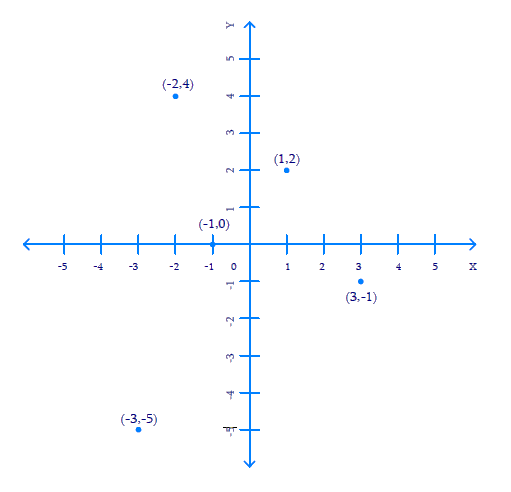# Ex.3.3 Q1 Coordinate Geometry Solution - NCERT Maths Class 9

Go back to  'Ex.3.3'

## Question

In which quadrant or on which axis do each of the points $$(–2, 4), (3, –1), (–1, 0), (1, 2)$$ and $$(–3, –5)$$ lie? Verify your answer by locating them on the Cartesian plane.

Video Solution
Coordinate Geometry
Ex 3.3 | Question 1

## Text Solution

Steps:

To determine the quadrant or axis of the points

$$(–2, 4), (3, –1), (–1, 0), (1, 2)$$

and $$(–3, –5)$$.

Plot the points

$$(–2, 4), (3, –1), (–1, 0), (1, 2)$$ and

$$(–3, –5)$$ on the graph, to getFrom the figure above, we can conclude that the points

• Point $$(–2, 4)$$ lie in $$2^\rm{nd}$$ quadrant
• Point $$(3, –1)$$ lie in $$4^\rm{th}$$ quadrant
• Point $$(–1, 0)$$ lie on the negative $$x-$$axis.
• Point $$(1, 2)$$ lie in $$1^\rm{st}$$ quadrant.
• Point $$(–3, –5)$$ lie in $$3^\rm{rd}$$quadrant.
Video Solution
Coordinate Geometry
Ex 3.3 | Question 1

Learn from the best math teachers and top your exams

• Live one on one classroom and doubt clearing
• Practice worksheets in and after class for conceptual clarity
• Personalized curriculum to keep up with school